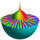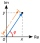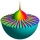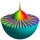# Complex numbers + multiplication - math problems

#### Number of problems found: 4

• Mistake in expressionWhile attempting to multiply the expression (2 - 5i)(5 + 2i) a student made a mistake. (2 - 5i)(5 + 2i) = 10 + 4i - 25i - 10i2 = 10 + 4(-1) - 25(-1) - 10(1) = 10 - 4 + 25 - 10 = 21 Complete the explanation and correct the error. Hint: The student inc
• Cis notationEvaluate multiplication of two complex numbers in cis notation: (6 cis 120°)(4 cis 30°) Write result in cis and Re-Im notation.
• Linear combination of complexIf z1=5+3i and z2=4-2i, write the following in the form a+bi a) 4z1+6z2 b) z1*z2
• Let z1=x1+y1iLet z1=x1+y1i and z2=x2+y2i Find: a = Im (z1z2) b = Re (z1/z2)

We apologize, but in this category are not a lot of examples.
Do you have an exciting math question or word problem that you can't solve? Ask a question or post a math problem, and we can try to solve it.

We will send a solution to your e-mail address. Solved examples are also published here. Please enter the e-mail correctly and check whether you don't have a full mailbox.

Try our complex numbers calculator. Complex numbers - math problems. Multiplication Problems.